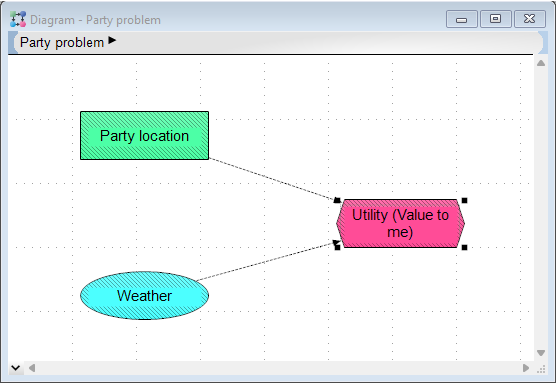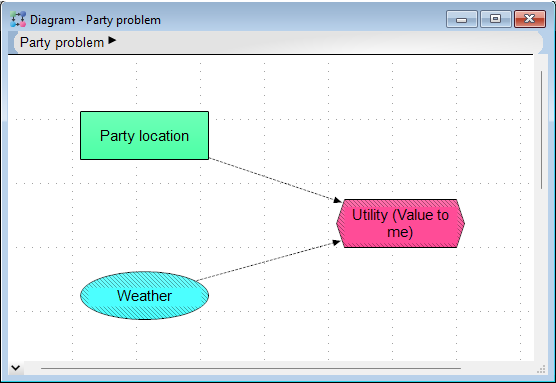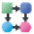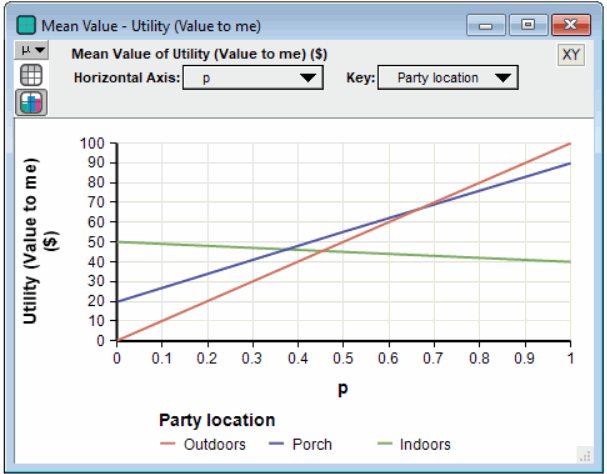# Tutorial: Decision trees

(diff) ← Older revision | Latest revision (diff) | Newer revision → (diff)

Release: 4.6  •  5.0  •  5.1  •  5.2  •  5.3  •  5.4  •  6.0  •  6.1  •  6.2  •  6.3  •  6.4

This chapter shows you how to:

• Define a variable as a probability table
• Define a variable as a deterministic table

In this chapter you will create a new Analytica model called Party Problem. (For information about how to create a new model, see the beginning of Tutorial: Create a model.) The Party Problem model illustrates the use of discrete probability distributions.

You should study this chapter if your models use discrete or conditional probabilities.

In many kinds of models, your variables can be described using probability distributions based on expert judgment or on empirical data. On those occasions when the outcomes are discrete or qualitative (for example, low, medium, or high), you might need to use discrete probability distributions.

In the Party Problem model, the key uncertain variable is weather: it could be sunny or rainy. The weather has an impact on the decision about the location of a party — indoors, on a porch, or outdoors.

## Documenting the model

Specify the information shown in this model’s Object window.1. Tab to or click in the Title field and type Party problem.
2. Tab to or click in the Description field and type the text shown here.

The Object window should now look similar to this:

## Creating the Party Location, Weather, and Utility variables

Using the techniques introduced in Tutorial: Creating variable nodes, you will create a Party Location decision variable, a Weather chance variable, and a Utility objective variable.

1. Create a Decision node and title it Party location.
2. Create a Chance node and title it Weather.
3. Create an Objective node and title it Utility (Value to me).

Your diagram should now look like this:

## Drawing arrows between variables

In this section, you will draw arrows from Party Location and Weather to Utility using the techniques introduced in Tutorial: Connecting multiple arrows.

Your diagram should now look like this:## Defining Party Location as a list of labels

Consider that there are three possible locations where you could hold your party — indoors, on the porch, and outdoors. You will define Party Location as a list of labels identifying each location.

Party Location is used to index the Utility (value to me) objective node, so it is similar to the Car Type index variable created for the Car Cost model in the section Tutorial: Creating an index variable. (Party Location is a decision variable, rather than an index variable, because it is directly under your control.)

1. Double-click Party Location to open its Object window.

2. Click in the Description field and type the text shown here.

3. Select List of Text from the Expression pop-up menu.

4. Click in the first cell and type Outdoors, then press the Enter key.
5. In the next cell, type Porch, then press the Enter key.
6. In the next cell, type Indoors, then press Tab to accept the changes.

The diagram should now look like this.## Defining Weather as a probability table

In this model, as in real life, weather is unpredictable.

In this section, you will characterize weather as having two possible discrete outcomes, either sunny or rainy. In addition, you will assign probabilities for each possible outcome. This is done by defining weather as a probability table. A probability table is Analytica’s function for describing discrete probabilities.

2. Click in the Description field and type the text shown here.
3. Select Probability Table from the Expression popup menu.

The Indexes dialog box opens to confirm your choice of index for the table. Weather (Self) appears in the Selected Indexes list. Self indicates that the index — the possible outcomes of the discrete distribution — is contained within the probability table. Self is required as an index of a probability table.

4. Click the OK button.

An Edit Table window appears. In this table, the first column holds the outcomes and the second column holds their probabilities. You will enter the possible outcomes in the first column.

5. Click in the cell labeled item 1 to select the first label for Weather. Type Sunny into the cell, replacing item 1, and press the Enter key.

A second row should be added to the table, containing the same label as the first row, Sunny. If a second row does not appear, make sure you have the text for Sunny selected, and press the Enter key again.

6. A second row is added to the table. Type Rainy and click in the first body cell to accept the entry.

In the second column, you will enter the probabilities of the possible outcomes.

7. Enter the values 0.4 and 0.6 for the probabilities of sunshine and rain.
8. Check the check buttonto accept these entries.

The mid value is Rainy, since more than 50% of the probability was assigned to Rainy.

## Defining Utility as a deterministic table

The utility of a party location decision depends on the outcome of the weather.

In this section, you will define Utility as a deterministic table (or determtable) using both Party Location and Weather.

A deterministic table appears similar to an edit table or probability table. At least one index of a DetermTable must be a discrete probabilistic variable (probability table). The result of evaluating a deterministic table takes into account the probability distribution described by the probability table index.

2. Enter the units and description as shown here.
3. Select Other from the Expression menu.

Because the Utility variable hasn’t been defined yet, the first function abs() in the first library Math is displayed. Libraries hold the functions available for you to use. For an overview of Analytica’s libraries and their functions, see the section Definition menu in Analytica User Guide.

You will select the DetermTable() function, which is in the Array library under -Table Functions, so click this to see it's contents...

4. Select Table functions from the Array library.
Tip

The Object Finder dialog a brief description of the selected function.

5. Scroll down the list and select Determ table.
6. To specify the indexes for the table click the indexesbutton.

Party Location and Weather are already selected as indexes because you drew arrows from them to Utility and they are defined as lists.

7. Click the OK button to accept these indexes.

Press OK to finish the Object Finder dialog, and an Edit table window opens for you to specify the dollar values for Utility.

You will give a value for each combination of party location and weather. These values describe the amount of money it is worth to you if you use a given party location and the weather turns out a certain way. Nominally, it is most valuable to have the party outdoors if it is sunny; it is least valuable if it is rainy and you are outdoors. Other values fall between these extremes.

10. Click the Diagram buttonto bring the Diagram window to the front.
8. Enter the values shown in the cells
9. Click the check button to accept these entries

## Computing Utility

In this section, you will compute the value of Utility.

The utility of each party location corresponds to the values you just entered for Rainy in the Utility deterministic table, because the mid value of Weather is Rainy. With these outcomes, a deterministic assessment leads you to conclude that your best bet is to hold the party indoors.

The mean value gives you a very different assessment: the porch gives you the greatest expected utility. The mean value as estimated by the sample is approximately 40 for outdoors, 48 for the porch, and 46 for indoors.

The exact expected utility for each party location can be calculated by multiplying the probabilities of the outcomes by the values of Utility:

Outdoors= (100)*0.4 + (0)*0.6 = 40
Porch= (90)*0.4 + (20)*0.6 = 48
Indoors= (40)*0.4 + (50)*0.6 = 46


### Note to those with a background in discrete modeling

Analytica simulates all probability distributions and calculates the expected (mean) value of a distribution by computing the average of the samples. For a discrete distribution, the computed mean converges, with increasing sample size, toward the value obtained by multiplying the probabilities by the discrete outcome values.

## Summary

In this chapter, you have:

• Defined a variable as a probability table, a discrete distribution.
• Defined a variable as a deterministic table, a function that defines a conditional dependency on the outcome of a discrete uncertain variable.

### Exercise

As an exercise, extend the model to examine how the utility of each party location varies as the probability of rain varies from 0 to 100%.

1. Create another chance node, titled p, the probability of sunshine. Define it as the range of probabilities from 0 to 100%, as Sequence(0, 1, 0.5).
2. Redefine the probabilities for Weather as p, for sunny, and (1- p), for rainy.
3. Recalculate the mean value of Utility. Display the result as a graph.